Pandas Query Examples: SQL-like queries in dataframes

# Pandas Query Examples: SQL-like queries in dataframes

Last updated:

Pandas v1.x used. See all examples on this jupyter notebook

### Why use query

Because it enables you to create views and filters inplace.

It is also faster than pure python for numerical operations.1

import pandas as pd

# using filters needs two steps
# one to assign the dataframe to a variable
df = pd.DataFrame({
'name':['john','david','anna'],
'country':['USA','UK',np.nan]
})

# another one to perform the filter
df[df['country']=='USA']


But you can define the dataframe and query on it in a single step (memory gets freed at once because you didn't create any temporary variables)

# this is equivalent to the code above
# and uses no intermediate variables
pd.DataFrame({
'name':['john','david','anna'],
'country':['USA','UK',np.nan]
}).query("country == 'USA'")


## Python variable

To reference external variables in the query, use @variable_name:

import pandas as pd
import numpy as np

df = pd.DataFrame({
'name':['john','david','anna'],
'country':['USA','UK',np.nan],
'age':[23,45,45]
})

target_age = 45

df.query('age == @target_age')


## OR operator

Just use or. Don't forget parentheses.

import pandas as pd

df = pd.DataFrame({
'name':['john','david','anna'],
'country':['USA','UK', 'USA'],
'age':[23,45,45]
})

df.query("(name=='john') or (country=='UK')")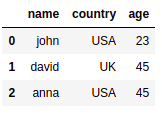Before: Source dataframe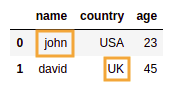After: only rows where name is 'john'
or country is 'UK'

## AND operator

Just use and. Don't forget parentheses.

import pandas as pd

df = pd.DataFrame({
'name':['john','david','anna'],
'country':['USA','UK', 'USA'],
'age':[23,45,45]
})

df.query("(country=='USA') and (age==23)")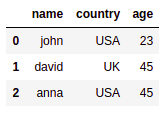Source Dataframe with all rows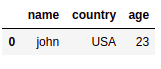After: only one row has
country='USA' and
age =23

## Multiple Conditions

See and operator and or operator above for more examples

• Example: AND operator

df.query((col1 == 1) and (col2 == 2))

• Example: OR operator

df.query((col1 == 1) or (col2 == 2))

## Value in array

Put values in a python array and use in @myvar:

import pandas as pd

df = pd.DataFrame({
'name':['john','david','anna'],
'country':['USA','UK', 'USA'],
'age':[23,45,45]
})

names_array = ['john','anna']

df.query('name in @names_array')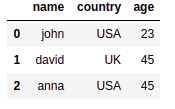Source dataframe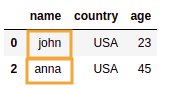Rows where name in ['john', 'anna']

## Not in array

Put values in a python array and use not in @myvar:

import pandas as pd

df = pd.DataFrame({
'name':['john','david','anna'],
'country':['USA','UK', 'USA'],
'age':[23,45,45]
})

invalid_array = ['anna']

df.query('name not in @invalid_array')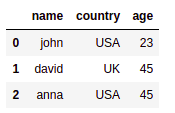Source dataframe: all rows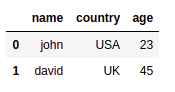Selected rows where name not in ['anna']

## Escape column name

To escape special characters such as whitespace, wrap column names in backticks: ''

df = pd.DataFrame({
'name':['john','david','anna'],
'country of birth':['USA','UK', 'USA'],
'age':[23,45,45]
})

df.query('country of birth == "UK"')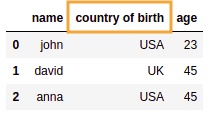Source dataframe: one of the column
names has spaces in it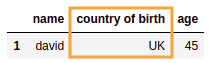Selected rows where
country of origin equals 'UK'

## Is null

note the use of engine='python'

To filter the dataframe where a column value is NULL, use .isnull()

import pandas as pd
import numpy as np

df = pd.DataFrame({
'name':['john','david','anna'],
'country':['USA','UK',np.nan]
})

df.query('country.isnull()', engine='python')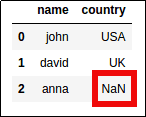Original Dataframe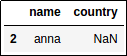Equivalent to:
"where country is NULL"

## Is not null

note the use of engine='python'

Use .notnull()

import pandas as pd
import numpy as np

df = pd.DataFrame({
'name':['john','david','anna'],
'country':['USA','UK',np.nan]
})

df.query('country.notnull()', engine='python')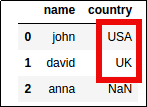Original Dataframe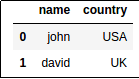Equivalent to:
"where country is NOT NULL"

## Like

Although like is not supported as a keyword in query, we can simulate it using col.str.contains("pattern"):

import pandas as pd

df = pd.DataFrame({
'col1':['foo','bar','baz','quux']
})

df.query('col1.str.contains("ba")')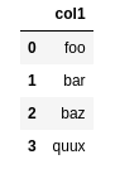Source dataframe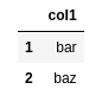Result: filter where col1 matches "ba"

## TypeError: unhashable type: 'Series'

Pass engine="python" as parameter:

df.query("....", engine="python")
`

1 It uses numexpr under the hood: https://github.com/pydata/numexpr